• https://zhuanlan.zhihu.com/intelligentunit 长期原创和翻译深度学习深度增强学习等领域高质量文章的专栏
https://zhuanlan.zhihu.com/intelligentunit
长期原创和翻译深度学习和深度增强学习等领域高质量文章的专栏
展开全文• ## 深度增强学习综述

千次阅读 2018-10-15 14:01:39
本文是对《深度增强学习综述》一文的解读。文章从增强学习谈起，谈到对深度学习的引入，层层递进、简明扼要地从技术层面阐释了深度增强学习的原理和效用。 当今世界，科技发展日新月异。即便是业界中人，往往也搞不...
本文是对《深度增强学习综述》一文的解读。文章从增强学习谈起，谈到对深度学习的引入，层层递进、简明扼要地从技术层面阐释了深度增强学习的原理和效用。

当今世界，科技发展日新月异。即便是业界中人，往往也搞不清同行们的动态。所以，隔三差五需要有人梳理业界的动态。

“Deep ReinforcementLearning: An Overview”  这篇论文的作者，梳理了深度学习与增强学习相融合的最新动态。

这是一篇苦心之作，

梳理了该领域各个学派的基本思路及其区别。

罗列了各种测试平台，目前主要是各种游戏，之所以用游戏来做测试，是因为容易采集数据。

值得读的论文，罗列了各个学派的重要论文。

枚举了若干重要的应用，包括游戏，重点介绍了下围棋的 AlphaGo，教无人机做特技，教机器人行走和跳跃，机器翻译和对话，智能医疗诊断，深度学习系统的自动设计等等。

论文：深度强化学习概览

作者：Yuxi Li，加拿大阿尔伯塔大学（University of Alberta）计算机系的博士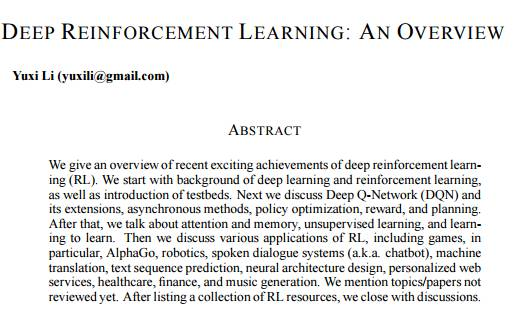摘要

本文概述了最近取得许多令人振奋的成果的深度增强学习（RL）。我们从深度学习和增强学习的背景开始说起，并介绍了各种测试平台。然后我们讨论了 Deep Q-Network（DQN）及其扩展，异步方法，策略优化，激励，以及规划。接着，我们讨论了注意力和记忆，无监督学习，以及学习学习（learning to learn）。我们还讨论了 RL 的各种应用，包括游戏（尤其是 AlphaGo），机器人，语音对话系统（即，chatbot），机器翻译，文本序列预测，神经架构设计，个性化网络服务，医疗保健，金融，以及音乐自动生成等。我们也提了一些尚没有综述的主题/论文。本论文以一系列值得阅读的 RL 重要论文的列举结束。

什么是增强学习，为什么需要引入深度学习？

不妨把机器学习看成二维空间，第一个维度是问题，第二个维度是方法，用什么方法来解决问题。

大致来说，机器学习想解决两大类问题，模拟和决策。

1. 模拟：

给定一系列输入 x 和输出 y，模拟两者之间的函数关系 y = f(x)。

预测是函数模拟的一个小类，譬如根据各种经济因素 x，预测股票价格。

分类是另一个小类，譬如给定一张人像照片 x，识别照片中的人物是不是y。

2. 决策：

先构造三元组，包括状态 state、行动action、收益 reward。

序列是一连串三元组

输入若干序列，作为训练数据。

决策的任务，是分析这些训练数据，发现最优行动策略，目的是获得最大的收益。

类似于金融折现率，未来的收益，折算成现钞，得打个折扣。远期的折扣，比近期的折扣大，这就是的意义。

决策的应用很多，下棋、开车、问答、医疗诊断、机器人自主行走跳跃等等，都是决策问题。

逻辑回归 Logistic Regression、向量机 SVM、决策树Decision Tree 等等是传统的机器学习的方法，主要用于解决模拟问题。

深度学习 Deep Learning 是最新的方法，起先也主要用于解决模拟问题。

增强学习 Reinforcement Learning，譬如 Q-learning 算法，是解决决策问题的传统方法。

为什么要用深度学习这个新方法解决决策问题？

目的无非是，1. 解决更复杂的决策问题，2. 学得更准，3. 学得更快，4. 用更少的训练数据。

传统的增强学习存在什么问题？

卢浮宫馆藏美术作品众多，经验丰富的导游，会帮游客规划一条参观路径，走最短的路，看最多的名画。

如何规划最佳参观路径，是一个决策问题。传统的方法，是增强学习 Reinforcement Learning。

简单而蛮力的办法，是找一张卢浮宫地图，尽可能罗列所有可能的参观路径，评价每一种路径的总收益。

这是 Monte Carlo tree search (MCTS) 的思路。

MCST 简单有效，但是缺陷是计算成本高。

下围棋的 AlphaGo，用 MCST 算法来精算局部的对弈策略。

但是，不能用这个方法来学习整盘棋的策略，因为如果要穷举整盘棋的所有对弈策略，数量级大概是。单机搞不定，云计算也搞不定。

如何降低计算量？思路是不必浪费精力去为槽糕的路径评估收益。问题是，如何甄别槽糕的路径？

不妨换一个思路。不会有观众故意跟自己过不去，专挑路程长、名画少的槽糕路径去参观卢浮宫。

我们不必关心哪条路径是槽糕的路径，我们只需要研究其他观众曾经走过的路径，就不会偏离最佳的路径太远。

什么是最佳路径？两个要素，

1. 当前收益大：每走一步，多半能看到一副名画。

2. 潜在的未来收益大：每走一步，离其它名画更近。

用数学公式来表达，就是，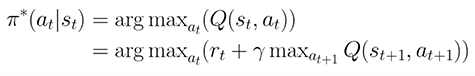的专业名称是行动价值Action Value。

用价值取代收益，既着眼当前收益，又兼顾未来潜力。

即便当前没有收益，譬如爬楼梯时一幅作品都看不到，但是楼上的展厅有名画，未来潜力巨大，爬楼就是明智的行动。

为了估算当前的行动价值，先得估算下一步的潜在价值。

为了估算下一步的潜在价值，先得估算再下一步的潜在价值。

重复以上步骤，直到走出卢浮宫出口。这个办法，就是 Q-learning算法。

Q-learning 算法是传统增强学习中最重要的算法。除此之外，有若干变种。

但是无论哪一种变种，面对复杂的问题，传统的增强学习算法都难以胜任。

如果状态不仅仅是位置，而且也包含每幅名作附近的拥堵程度，参观卢浮宫的路径规划就变得更复杂了。

如果行动不仅仅是前进、后退、左转、右转，而且也包括速度，问题就更复杂了。

如果收益不仅仅取决于作品的知名度，而且也包括观众的品味，问题就更复杂了。

解决复杂的决策问题，是增强学习与深度学习融合的一大动机。

增强学习与深度学习融合的切入点，是用结构复杂的神经网络，来估算增强学习中的行动价值、收益、策略等等。

深度增强学习深在哪里？

1. 用深度学习来估算价值

Deep Q-network (DQN)， Double DQN ， Dueling architecture 等等算法，都是这个思路。

先根据训练数据，来计算当前的行动价值，

然后用深度神经网络，估算，如果估算得完全准确，那么

。

如果两者之间有差距，那么就不断调整深度神经网络的参数，缩小两者的差距，这就是深度增强学习的训练过程。

这个办法，就是 DeepQ-network DQN，及其变种 Double DQN 的基本原理。

DQN 的计算成本较大，为了学得更快。决斗模型 Dueling architecture 提议，拆解。

把拆解为，其中是每个状态的价值，而是指在状态下采取行动后，价值的增量。

DQN 算法用一个规模较大的神经网络，去模拟，训练过程的计算成本高。

而决斗架构 Duelingarchitecture 用两个规模较小的神经网络，分别去模拟和，从而大大降低了训练过程的计算成本。

2. 用深度学习来估算策略

DPG (Deterministic PolicyGradient)，Deep DPG，GPS (Guided Policy Search)，TRPO (Trust Region Policy Optimization) ，PGQ 等等算法，都是这个思路。

DPG 把增强学习的训练过程，类比成演员和评委的互动。

DPG 用一个神经网络 actor，去模拟策略。

用另一个神经网络 critic，评估使用某种策略后的行动价值。

选定了策略 π 后，在每一种状态，actor 都会选择固定的行动，所以，

。

Deep DPG 在此基础上，做了一些改进。尤其是把训练数据回放，并且每次训练时，柔和地改进网络参数。

回放和柔和改进，目的是为了既不增加训练数据的数量，又提高训练的稳定性。

3. 用深度学习来估算收益

某些应用场景的收益，无法用简单的方式表达，譬如模仿书法家的字体风格，或者模仿某位飞行员的特技飞行动作。

反向增强学习 Inverse ReinforcementLearning，小样本学习 One-shot Learning 等等算法，针对这类模仿问题，做过有益的尝试。

GAN 主要由两个神经网络构成，

1. 生成模型Generator，从训练数据中猜测这些样本的数据分布，并伪造数据。

2. 鉴别模型Discriminator，识别哪些数据是真实的训练数据，哪些是生成模型伪造的假数据。

两个模型不断对抗，使得生成模型伪造的数据越来越像真的，而鉴别模型的识别能力越来越精准。

对抗的结果，是生成模型对数据分布的猜测，越来越精准。

模仿，是增强学习的一个子领域。

把 GAN 应用于增强学习，将是一大热点。

4. 融合

DQN 用深度学习来估算价值函数，DPG 用深度学习来估算策略函数，GAN 用深度学习来估算难以言说的收益函数。

Differentiable NeuralComputer (DNC) 不纠结价值函数和策略函数的细节，而是把它们看成黑盒子的内部构成。

DNC 用 LSTM 来分析训练数据，把分析结果保存在 LSTM 外部的存储空间。

LSTM 分析结果是一组数字向量，数字向量的物理意义很隐晦，如同表达生命特征的 DNA，但是它们包含了和的内容。

虽然数字向量的物理意义很隐晦，但是只要黑盒子能够做出正确的决策，就是好盒子。

DNC 的论文作者，用这个技术来玩积木游戏，来规划伦敦地铁任何两站之间的最佳换乘路径，效果很好。

黑盒子能不能完成更复杂的任务？这是吊足观众胃口的悬念。
展开全文• 一、背景深度学习的兴起让增强学习这个古老的机器学习分支迎来一轮复兴。它们的结合领域-深度增强学习（Deep ...之前两篇杂文深度增强学习（DRL）漫谈 - 从DQN到AlphaGo和深度增强学习（DRL）漫谈 - 从AC（Actor-Critic
一、背景
深度学习的兴起让增强学习这个古老的机器学习分支迎来一轮复兴。它们的结合领域-深度增强学习（Deep reinforcement learning, DRL）随着在一系列极具挑战的控制实验场景及其它跨领域的成功应用，现在已是各大顶级AI会议的热门topic之一。之前两篇杂文《深度增强学习（DRL）漫谈 - 从DQN到AlphaGo》和《深度增强学习（DRL）漫谈 - 从AC（Actor-Critic）到A3C（Asynchronous Advantage Actor-Critic）》  瞎侃了一些当前流行的DRL方法（主要由DeepMind主导）。这篇文章再来聊一下另一个相对独立的方法分支（主要由OpenAI主导）。近年来，学界开始将优化方法中的信赖域（Trust region）方法引入增强学习，并在各种实验场景中取得了良好的效果。其中典型的有TPRO和受其影响衍生出的一系列前沿算法，如PPO，Trust-PCL，ACER等。其中PPO已成为OpenAI的默认增强学习算法。
这里准备聊一下它们的前生今世。信赖域系增强学习方法，顾名思义，来源于优化论中的信赖域方法和机器学习中增强学习的结合。那咱们先话分两头聊下这两部分，先从信赖域方法开始。在Jorge Nocedal和Stephen J. Wright的《Numerical Optimization》一书的第2.2节介绍了解优化问题的两种策略-line search和trust region。本质上它们的作用都是在优化迭代过程中从当前点找寻下一点。它们的最大区别是先确定步长还是先确定方向。Line search方法先确定方向再确定步长。而trust region方法则先把搜索范围缩小到一个小的范围，小到能够用另一个函数（Model function）去近似目标函数（Objective function），然后通过优化这个model function来得到参数更新的方向及步长。在该书的第三和第四章分别着力介绍了line search和trust region方法，感兴趣可以进一步了解。
另一头我们从增强学习中的策略梯度（Policy gradient, PG）方法说起。PG方法是RL中非常重要而又历史悠久的一类方法。早在1992年Ronald J. Williams提出的REINFORCE算法就是PG的雏形。它的基本思想是考虑由参数$\theta$控制的随机策略$\pi(\theta)$，然后通过优化与策略相关的目标函数$J(\theta)$（比如累积折扣回报和）来更新策略的参数。这种policy-based方法与RL中另一个重要分支value-based方法相比，不仅避免了值函数（Value function）误差导致的策略退化（Policy degradation），而且更加易于用在连续动作空间问题。但是它也有一些缺点：其一是数据效率（Data efficiency）或是说样本利用率低；其二是方差（Variance）大，它会使得训练困难基于无法收敛。针对这些缺点，其中一个比较经典而有效的方法是在原始PG方法中引入value-based方法对值函数进行估计，这就是行动者-评论家（Actor-Critic, AC）方法，同时在估计的值函数减去baseline来减少variance。但是，AC算法在策略搜索过程中如果碰到大的平坦区域（Plateau）就会导致收敛速度过慢，或者过早收敛（当然这是基于梯度算法的通病）。原因是这些地方梯度很小，或不直接指向最优解。Amari在1998年的论文《Natural Gradient Works Efficiently in Learning》中讨论了通过自然梯度（Natural gradient）代替标准梯度（Standard gradient）来解决这个问题。当参数坐标系统不为正交，参数空间为黎曼空间（比如神经网络中）。这时目标函数的最速下降方向定义为在参数变化测度为固定值下最小化目标函数的参数变化方向。这个方向可表示为$G^{-1}(\theta) \nabla_\theta J$，其中的$G$称为黎曼度量张量（Reimannian metric tensor）。考虑统计估计问题时，参数空间的黎曼结构为Fisher information matrix(FIM)。其形式为$F=\mathbb{E}_{\theta} [ \nabla_{\theta} \log P_{\theta}(X) \nabla_{\theta} \log P_{\theta}(X) ^T ]$。它与KL散度（Kullback-Leibler divergence）有密切关系。具体可以参见KL散度的Wiki中的Fisher information metric一节。对于参数$\theta^*$及其邻域的一点$\theta$，一方面$D_{KL}(\theta^*, \theta)=0$，另外由于当$\theta = \theta^*$时KL散度达到最小，因此KL散度相对于参数的一阶导数为0，综上用泰勒展开到二阶只剩下二次项，而该二次项中的Hessian矩阵（半正定）即为Fisher information matrix（FIM）。直观上，它代表着相应参数下似然的梯度的变化率。如果要参数更新前后其表征分布间的KL距离为定值，可以将FIM替代上面的度量张量，最速下降方向就由传统梯度$\nabla_\theta J$变为$F^{-1}_\theta \nabla_{\theta} J$，即FIM的逆乘以标准梯度。直观上，它使得策略在相对于参数不太敏感的地方，步长大；而在敏感的地方，步长小。当参数空间为欧式空间时，自然梯度退化成标准梯度。
有了这些背景知识后，下面从几篇经典论文中一窥其发展历程。这些方法之间关系大体如下：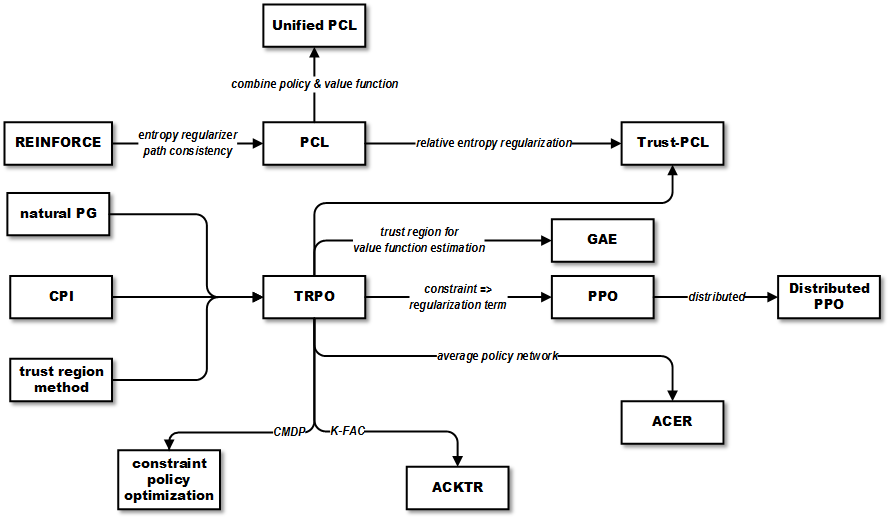二、相关论文选读
《Trust Region Policy Optimization》 Schulman, J., Levine, S., Moritz, P., Jordan, M. I. & Abbeel, P. 2015
Kakade在2002年的论文《Approximately Optimal Approximate Reinforcement Learning》中提出了conservative policy iteration(CPI)方法，该方法在一定的前提下（restart distribution和approximate greedy policy）能找到近似的最优策略。定义策略性能（Policy performance）为期望累计折扣回报，记为$\eta$。策略更新前后的策略性能之差可用优势函数（Advantage function）的折扣累加期望表示：$\eta(\tilde{\pi}) = \eta(\pi) + \mathbb{E}_{s_0, a_0,... \sim \tilde{\pi}} [\sum^{\infty}_{t=0} \gamma^t A_{\pi} (s_t, a_t)]$。它表示如果策略更新后所有状态的优势函数值为非负，则策略性能一定增长。为了避免状态稳态分布对于更新后策略的依赖导致难以优化的问题，这里引入了策略性能的一阶近似$L_{\pi}(\tilde{\pi})$。CPI方法中以下面这种mixture policy的方式更新策略$\pi_{new} = (1-\alpha) \pi_{old} + \alpha \pi'$，并且给出了在这种更新方式下策略性能的增长的下界（定理4.1）。而《Trust Region Policy Optimization》一文中将这个结论从mixture policy拓展到一般的随机策略。具体做法是将$\alpha$用total variation divergence在各状态下的最大值$D^{\max}_{TV} (\pi_{old}, \pi_{new})$替代，另外考虑total variation divergence和KL散度的关系$D_{TV}(p || q)^2 \leq D_{KL}(p || q)$，可以得到更新后策略性能的下界为：
$L_{\pi}(\tilde{\pi}) - CD^{\max}_{KL} (\pi, \tilde{\pi})$
其中$C=\frac{2\epsilon \gamma}{(1-\gamma)^2}$，$\epsilon=\max_s|\mathbb{E}_{a \sim \pi'(a|s)} [A_\pi (s,a)]|$。因此问题就转化为最大化这个下界。这个下界也称为原目标函数的代理函数（surrogate function）。
实际中，直接优化这个代理函数会导致步长非常小，TRPO的做法是用前后策略的KL散度的约束（即trust region constraint）$D^{\max}_{KL}(\theta_{old}, \theta) \leq \delta$来代替上面的惩罚项。但由于约束太多，这里用平均KL散度$\bar{D}^{\rho}_{KL}$代替。这样优化问题就变为在$\bar{D}^{\rho}_{KL}(\theta_{old},\theta) \leq \delta$约束下最大化目标函数$L_{\theta_{old}}(\theta)$。估计$L_{\theta_{old}}$时采用importance sampling，采样分布为$q(a|s) = \pi_{\theta_{old}}(a|s)$。文中给出两种采样方法-single path和vine。后者与前者相比，它会在轨迹中的每个状态中执行不同动作。它的好处是variance低，但缺点是模拟的effort较大。
$\max_{\theta} \quad L_{old}(\theta) \\ s.t. \quad \bar{D}_{KL}(\theta_{old}, \theta) \leq \delta$
为了计算参数更新方向，先对约束中的KL散度作二次近似$\bar{D}_{KL} \approx \frac{1}{2} (\theta - \theta_{old})^T F (\theta - \theta_{old})$，其中$F$为FIM。和natural policy gradient一样，参数更新方向为自然梯度$F^{-1} g$。因为像神经网络表示策略时会有上千参数，这里如果要生成和求FIM的逆计算量会非常大，因此这里采用conjugate gradient算法近似求解。这称为Truncated Natural Policy Gradient(TNPG)方法，它与TRPO区别在于它没有像TRPO一样强制满足约束。接下来TRPO采用line search方法在前面选取的方向上再选取步长保证约束被满足。
《Proximal Policy Optimization Algorithms》 Schulman, J., Wolski, F., Dhariwal, P., Radford, A. & Klimov, O. 2017
PG方法的缺点是数据效率和鲁棒性不好。同时TRPO方法又比较复杂，且不兼容dropout（在深度神经网络训练过程中按照一定概率对网络单元进行丢弃）和参数共享（策略和值函数间）。这篇论文提出了PPO算法，它是对TRPO算法的改进，更易于实现，且数据效率更高。TRPO方法中通过使用约束而非惩罚项来保证策略更新的稳定性，主要原因是作为惩罚项的话会引入权重因子，而这个参数难以调节。TRPO中为了解优化问题，先线性近似目标函数，二阶近似约束，最后通过conjugate gradient算法和line search求解。而这篇文章尝试通过一阶优化的方法来解。与TRPO中用约束来限制策略更新幅度不同，PPO中采用了惩罚项，或者说正则项的做法。文中提出了基于clipped probability ratio的目标函数。首先定义probability ratio $r_t(\theta) = \frac{\pi_\theta (a_t|s_t)}{\pi_{\theta_{old}}(a_t|s_t)}$，TRPO的代理目标函数，同时也是CPI方法中的目标函数可表示为$L^{CPI}(\theta) = \hat{\mathbb{E}}_t [r_t(\theta) \hat{A}_t]$。这样的目标下策略更新有可能会很大。因此，clipping目标函数中加入了clip项:
$L^{CLIP}(\theta) = \hat{\mathbb{E}}_t [\min(r_t(\theta) \hat{A}_t, \text{clip}(r_t(\theta), 1 - \epsilon, 1+\epsilon) \hat{A}_t)]$
其中$\epsilon$为超参数。其中的clip项促使得$r_t$不偏离$[1-\epsilon, 1+\epsilon]$所定义的区间。其中会对clipped和unclipped目标进行最小化操作，意味着它是unclipped目标的下界。直观上，当策略更新的偏移超出预定区间而获得更大的目标函数值时，这个clip项就会产生影响。
另外一种目标函数是采用自适用的KL惩罚项系数，虽然实验中效果不及上面clipping目标函数。它的目标函数为：
$L^{KLPEN}(\theta) = \hat{\mathbb{E}}_t[\frac{\pi_\theta(a_t|s_t)}{\pi_{\theta_{old}}(a_t|s_t)} \hat{A}_t - \beta \; \text{KL}[\pi_{\theta_{old}(\cdot|s_t), \pi_\theta(\cdot|s_t)}] ]$
当策略更新前后KL散度小于预定值时，惩罚项系数$\beta$减小到原来的一半；当大于预定值时，系数增加一倍。如果使用神经网络和共享策略和值函数参数的话，就需要在损失函数中结合策略优化目标和值函数误差项：
$L^{CLIP+VF+S}_t(\theta) = \hat{\mathbb{E}}_t [L^{CLIP}_t (\theta) - c_1 L^{VF}_t(\theta) + c_2 S[\pi_\theta](s_t)]$
其中的$c_1$和$c_2$为系数，$S$和$L^{LF}_t$分别为策略熵和值函数误差项。整个PPO算法每次迭代中，先通过执行当前策略估计优势函数，然后通过优化代理函数更新策略参数。
《Emergence of Locomotion Behaviours in Rich Environments》 Heess, N. et al. 2017
DeepMind在该论文中提出了分布式的PPO算法（DPPO）。如它的名字，它是对上面PPO算法的改进。很多的RL算法假设有well-defined的回报函数。但在很多场景中，尤其是连续动作空间控制问题中，这个假设并不成立。本文讨论的一个重点就是如何只利用简单的回报函数，通过丰富多变的环境来学习到稳定的行为。为了达到这个目的，需要改善增强学习算法的伸缩性。
我们知道，PG方法的缺点是variance高，且对超参数敏感。解决方法之一就是上面提到的trust region约束，TRPO就是基于该基本思想。PPO将trust region约束实现为正则项。而在DPPO算法中，数据的收集和梯度的计算被分布到多个worker中，思想类似于A3C算法。原始的PPO算法通过完整的回报和估计策略优势。而为了便于使用batch update的RNN，这里使用了K-step returns来估计策略优势。DPPO算法分为两部分-chief和worker。worker部分在每次迭代中依次做M步策略参数和B步值函数参数的更新。chief部分从worker收集梯度，收集指定个数后，将它们的均值更新到总的参数。对于每个worker，每轮迭代中按当前策略执行T步，然后把它们按K个数据一份分好，对于每K步样本，估计优势函数，然后分别通过梯度$\nabla_\theta J_{PPO}$和$\nabla_\phi L_{BL}$更新相应参数。另外它会根据当前策略和之前策略的KL距离是否超出区域调节目标函数中的系数$\lambda$。作者用TensorFlow实现了DPPO算法，参数位于参数服务器，workers在每次梯度更新后同步它们的参数。实验中将DPPO算法与TRPO和A3C算法在几个控制任务中的性能作了对比，显示比后两者有更好的表现，同时它还有很好的伸缩性。
《High-Dimensional Continuous Control Using Generalized Advantage Estimation》 Schulman, J., Moritz, P., Levine, S., Jordan, M. I. & Abbeel, P. 2016
PG方法中经常会采用神经网络作为非线性函数逼近器。但有两个问题：一是需要大量的样本；二是在数据并不稳定的情况下策略无法稳定更新。第一个问题可以用优势函数的指数加权估计（Exponentially-weighted estimator）解决。第二个问题可以用对策略和值函数（用神经网络表示）的trust region优化来解决。
PG是估计参数化概率策略的经典方法。PG方法中的每次更新应该提高那些能够好于平均的动作的概率，同时减少差于平均动作的概率。但是其variance很高，因此AC算法通过使用值函数代替经验回报（Empirical return）以引入bias为代价降低variance。但事实上bias有时往往更加有害。这篇论文主要讨论在控制bias的前提下大大减少variance。以$\gamma$和$\lambda$作为两个可调参数，提出了GAE（generalized advantage estimator）方法。PG中的梯度$g = \nabla_{\theta} \mathbb{E} [\sum^{\infty}_{t=0} r_t]$具体可以写成通用形式：
$g = \mathbb{E} [\sum^{\infty}_{t=0} \Psi_t \nabla_{\theta} \pi_\theta (a_t|s_t)]$
其中选择优势函数 $\Psi_t = A^{\pi} (s_t,a_t)$时variance最小。优势函数用于定量地表示一个动作好于还是坏于平均行为。因此该文主要精力也放在优势函数的估计。总得来说，GAE($\gamma$, $\lambda$)是k步估计的指数加权移动平均。
这里把discounted MDP中的折扣因子$\gamma$作为该模型中的用于减少variance的参数。另一个参数类似于TD($\lambda$)中的$\lambda$，用于不同k-step A函数估计的权重。这两个参数都可用于bias-variance之间的tradeoff。考虑这两个参数，优势函数的估计就是各k步估计的指数加权平均：
$\hat{A}^{GAE(\gamma, \lambda)}_t = \sum^{\infty}_{l=0} (\gamma \lambda)^l \delta^{V}_{t+l}$
这里的策略和值函数都是采用神经网络表示。和TRPO方法类似，这里也用了trust region方法。不同的是它除了用于策略更新，还用于值函数估计。
《Sample Efficient Actor-Critic with Experience Replay》 Wang, Z. et al.  2017
之前很多RL方法的成功需要大量模拟，也就是说它们是样本利用率不高。像DQN这样的基于值函数估计方法在这方面会好些，但DQN有自己的缺点，如不能用于连续动作空间。另外PG方法中经典的A3C算法也有样本利用率低的问题。因此，这篇文章的目标就是要设计稳定的数据效率高的AC方法并能应用于连续和离散的动作空间。本文引入了Actor critic with experience replay(ACER)算法，它和DQN的性能差不多，且比A3C在Atari实验场景中和连续控制领域的数据效率好很多。ACER算法引入很多创新点，如truncated importance sampling with bias correction, stochastic dueling network architectures和efficient trust region policy optimization。这里主要看下它对TRPO的改进。
TRPO算法中采用了conjugate gradient方法求解，会有很多Fisher-vector乘积运算。因此在大规模问题中计算量十分大。与TRPO方法通过约束条件限制策略更新，ACER算法中维护average policy network用于表示过往策略的滑动平均，并保持策略更新不偏离这个均值。这个average policy network用$\phi_{\theta_a}$表示。深度神经网络参数记为$\theta$，它的输出作为策略分布$\pi(\cdot|x) = f(\cdot|\phi_\theta(x))$的统计量。ACER的策略梯度记为$\hat{g}^{\text{acer}}_t$。引入trust region后，可转为约束问题：
$\min_z \frac{1}{2} || \hat{g}^{\text{acer}}_t - z ||^2_2 \\ s.t. \quad \nabla_{\phi_\theta} D_{KL} [f(\cdot|\phi_{\theta_a}(x_t)) || f(\cdot|\phi_\theta(x_t))]^T z \leq \delta$
注意该约束为线性，因此整个问题可转为二次规划问题，有解析解：
$z^* = \hat{g}^{\text{acer}}_t - \max \{ 0, \frac{k^T \hat{g}^{\text{acer}}_t - \delta}{||k||^2_2} \} k$
直观上，这个解表示在约束满足的情况下，则解即为$\hat{g}^{{acer}}_t$，如果不满足，则沿$k$方向减少。以上是第一阶段，第二阶段中使用反向传播（Back propagation, BP）算法，通过$\frac{\partial \phi_{\theta} (x)}{\partial \theta} z^*$更新网络参数$\theta$。和TRPO方法中用二次近似约束中的$D_{KL}$不同，这种方法将约束写为线性形式，因此可以通过解析解得到目标对于 $\phi_{\theta}$的梯度，然后通过NN更新$\phi_{\theta}$对于$\theta$的梯度。从而避免了Fisher-vector计算，因此更适合大规模问题。
《Constrained Policy Optimization》 Achiam, J., Held, D., Tamar, A. & Abbeel, P. 2017
有些场景下，我们需要限定约束防止灾难性后果。比如工业机器人或者飞行器控制，如果不加限制可能会导致经济损失甚至有安全隐患。文章提出Constrained Policy Optimization（CPO）方法，是一种可以保证在每一次迭代中满足约束的通用策略搜索算法。
基于CMDP（constrained Markov decision process）模型。该模型引入一组代价函数$C_1,...,C_m$，其中$C_i:S \times A\times S \rightarrow \mathbb{R} $。目标函数$J_{C_i}$代表以$C_i$为代价函数的策略$\pi$下的期望折扣回报。$\Pi_{C}$为对于所有$i$，满足$J_{C_i}(\pi) \leq d_i$的策略集合，那要找的最优策略就是在这个集合当中使目标函数最大的那个。基于TRPO，在CMDP下的策略搜索问题可以写成公式：
$\pi_{k+1} = \arg \max_{\pi \in \Pi_\theta} J(\pi) \\ s.t. \quad J_{C_i} (\pi) \leq d_i \quad i = 1,...,m \\ D(\pi, \pi_k) \leq \delta$
具体地，选定$D_{KL}$作为测度，即CPO算法。和TRPO一样，为了避免过小的更新，用约束替代惩罚项：
$\pi_{k+1} = \arg \max_{\pi \in \Pi_\theta} \mathbb{E}_{s \sim d^{\pi}k} [A^{\pi_k}(s,a)] \\ s.t. \quad J_{C_i}(\pi_k) + \frac{1}{1-\gamma} \mathbb{E}_{s \sim d^{\pi}k, a \sim \pi} [A^{\pi_k}_{C_i}(s,a)] \leq d_i \quad \forall i \\ \bar{D}_{KL}(\pi || \pi_k) \leq \delta$
当参数空间维度很大时，上式的计算量非常大。把目标函数和代价约束通过线性近似，KL散度约束通过泰勒二阶展开近似后，可得：
$\theta_{k+1} = \arg \max_\theta g^T(\theta - \theta_k) \\ s.t \quad c_i + b^T_i(\theta - \theta_k) \leq 0 \quad i = 1,...,m \\ \frac{1}{2}(\theta - \theta_k)^T H (\theta - \theta_k) \leq \delta$
该问题为凸优化，可通过对偶问题解决。先构造更易解的对偶问题，它是有解析解的（附录定理 2）。解完对偶问题，即可根据对偶问题的解构造原问题的解。但由于近似误差，可能解并不能满足约束了，因此，还需要进行回溯线搜索（Backtracking linesearch）保证满足约束。
《Bridging the Gap Between Value and Policy Based Reinforcement Learning》 Nachum, O., Norouzi, M., Xu, K. & Schuurmans, D. 2017
这篇论文中提出了Path Consistency Learning (PCL)算法。我们知道，最初RL算法分value-based和policy-based两大类。policy-based方法最大的缺点是效率低。另外策略梯度通过rollout来估计的话会导致variance很大。为了解决这个问题，AC算法通过值函数估计来替代rollout估计，以引入bias为代价减小了variance。尽管如此，如果这个值函数估计是on-policy的话仍然会面临样本数据效率的问题（因为要么用on-policy数据，要么更新足够慢以防止bias）。而off-policy算法（如Q-learning）现实使用中需要花很大力气进行超参数调节才能稳定。为了结合on-policy训练的无偏性和稳定性，和off-policy的数据效率，off-policy AC方法出现了，但它仍没有解决在有函数近似（Function approximation）下的off-policy学习的一些理论问题。在这个背景下，这篇文章研究了熵正则化下策略优化和softmax value consistency的关系，提出了一种稳定的off-policy学习方法。
首先假设策略是以神经网络定义的分布$\pi_{\theta}(a|s)$，Q-learning基于hard-max Bellman公式，通过hard-max Bellman时域一致性（Temporal consistency）来更新参数。定义$O_{\text{ER}}(s, \pi)$为期望折扣回报。因为最优策略是通过$\pi^*=\arg\max_{\pi}O_{\text{ER}}(s, \pi)$来计算，因此策略为one-hot分布。本文主要研究softmax temporal consistency。它在传统的期望回报目标函数中加了折扣熵正则项（Discounted entropy regularizer）。这个正则项可以帮助exploration，避免过早收敛到次优解。正则化后的期望回报可以写成：
$O_{\text{ENT}}(s,\pi) = O_{\text{ER}}(s,\pi) + \tau \mathbb{H}(s, \pi)$
其中$\mathbb{H}(s, \pi)$为折扣熵项（discounted entropy term）。当$\tau > 0$时，最优解不再是one-hot分布，最优解可以表示为：
$\pi^*(a|s) \varpropto \exp \{(r(s,a) + \gamma V^*(s')) / \tau\}$
文中定理1给出了对于任意状态和动作的时域一致性属性（temporal consistency property）。它可以被推广到多步情况下（即文中推论 2）：
$V^*(s_1) - \gamma^{t-1} V^*(s_t) = \sum^{t-1}_{i=1} \gamma_{i-1}[r(s_i, a_i) - \tau \log \pi^*(a_i|s_i)]$
即对于给定状态动作序列下的时域一致性。基于此，PCL的目标是对于给定子轨迹（Sub-trajectory），上式中等式左边和右边之差尽可以接近于0。设$\phi$和$\theta$分别为值函数和策略的参数，PCL的目标可表示为：
$O_{\text{PCL}} (\theta, \phi) = \sum_{s_{i:i+d} \in E} \frac{1}{2} C(s_{i:i+d}, \theta, \phi)^2$
其中$C(s_{i:i+d}, \theta, \phi)$为对于长度为$d$的子轨迹，上面推论中等式左右边之差。然后就可以用梯度更新参数了。它可适用于on-policy和off-policy策略。
如果将soft consistency error用Q函数来表示的话，$Q_{\rho}$以$\rho$为参数，则值函数和策略都可以用$Q$函数表示:
$V_{\rho}(s) = \tau \log \sum_a \exp {Q_\rho(s,a) / \tau} \\ \pi_{\rho}(a|s) = \exp {(Q_\rho(s,a) - V_{\rho}(s)) / \tau}$
它将策略和值函数结合成一个模型，然后只要通过梯度更新一个参数$\rho$即可。这称为Unified PCL（Unified Path Consistency Learning）。结果表示，与PCL相比Unified PCL方法在一些简单任务中表现不好，但在一些复杂任务中表现更好。
《Trust-PCL: An Off-Policy Trust Region Method for Continuous Control》 Nachum, O., Norouzi, M., Xu, K. & Schuurmans, D.  2017
多数的policy-based方法（如REINFORCE算法）的基本思想是先参数化策略然后最大化期望回报$O_{\text{ER}}(s, \pi)$。PCL给这个目标函数添加了折扣熵正则项 $\mathbb{H}(s,\pi)$。Nachum等人的论文中证明了$O_{\text{ENT}}$的最优策略$\pi^*$和最优目标值$V^*(s) = O_{\text{ENT}}(s, \pi^*)$之间对于任意状态动作序列符合softmax temporal consistency constraint。在TRPO方法中，为了避免传统policy-based算法（优化$O_{\text{ER}}$）中的稳定性问题，引入了当前策略下的每个状态下KL散度的平均作为测度的trust region。结合了这种思想，在PCL优化$O_{\text{ENT}}$的基础上，加上折扣相对熵依赖域（Discounted relative-entropy trust region），于是就有了下面的约束优化问题：
$\max _{\pi} \mathbb{E}_s[O_{\text{ENT}}(\pi)] \\ s.t. \quad \mathbb{E}_s [\mathbb{G}(s, \pi, \tilde{\pi}) \leq \epsilon]$
其中的折扣相对熵有递归定义：
$\mathbb{G}(s, \pi, \tilde{\pi}) = \mathbb{E}_{a, s'} [\log \pi(a|s) - \log \tilde{\pi}(a|s) + \gamma \mathbb{G}(s', \pi, \tilde{\pi})]$
注意目标函数中熵正则化与约束中的相对熵信赖域的区别。前者可以鼓励exploration，而后者可以提高稳定性，加快训练速度。通过引入拉格朗日乘子，可以将上面的约束问题转为非约束问题$O_{\text{RELENT}(s,\pi)} = O_{\text{ENT}}(s, \pi) - \lambda \mathbb{G} (s, \pi, \tilde{\pi})$。该式类似于$O_{\text{ENT}}$，可以分解为两部分$O_{\text{RELENT}}(s,\pi) = \tilde{O}_{\mathbf{s, \pi}} + (\tau + \lambda) \mathbb{H}(s, \pi)$，其中的$\tilde{O}_{\text{ER}}(s,\pi)$为变换后回报函数$\tilde{r}(s, a) = r(s,a) + \lambda \log \tilde{\pi}(a|s)$下的期望回报目标。该目标下的最优值函数记为$V^*(s)$，最优策略记为$\pi^*(a|s)$，然后在此基础上将PCL中的结论拓展过来，可以得到下面的softmax temporal consistency constraint：
$V^*(s_0) = \mathbb{E}_{r_i, a_i, s_i} [\gamma^d V^* (s_d) + \sum^{d-1}_{i=0} \gamma^i (r_i - (\tau + \lambda) \log \pi^*(a_i|s_i) + \lambda \log \tilde{\pi}(s_i|s_i))]$
类似地，定义一致性误差（Consistency error） $C(s_{t:t+d}, \theta, \phi)$为上式等式左右边之差，我们的目标就是对于每个子轨迹使这个一致性误差的平方最小化，因此可以用梯度下降法更新策略和值函数的参数$\theta$和$\phi$。这就是trust-PCL的主要精神。
Trust-PCL比TRPO算法更加易于实现，它只需要简单的梯度下降。同时实验中发现Trust-PCL在一些控制任务中在平均回报和样本效率上优于TRPO。
《Scalable trust-region method for deep reinforcement learning using Kronecker-factored approximation》 Wu, Y., Mansimov, E., Liao, S., Grosse, R. & Ba, J. 2017
最近提出的Kronecker-factored方法可用于近似曲率矩阵。这篇文章扩展了natural policy gradient框架，利用Kronecker-factored approximate curvature(K-FAC)方法优化AC方法中的actor和critic，即策略和值函数的估计，提出了Actor Critic using Kronecker-Factored Trust Region(ACKTR)方法。
前面提到自然梯度可以用于提高样本利用率。但计算自然梯度需要计算FIM的转置，这是计算量非常大的操作。TRPO中使用conjugate gradient descent替代FIM的存储和求逆操作，只需要求FIM和向量的积。但它需要在每次参数更新时迭代求解。这阻碍了TRPO应用在大规模问题中。James  Martens和Roger Grosse在论文《Optimizing Neural Networks with Kronecker-factored Approximate Curvature》中提出了神经网络中的自然梯度近似方法K-FAC（Kronecker-factored Approximate Curvature），它可以显著减少计算量。ACKTR算法将之应用到AC算法的actor和critic中。实际中，ACKTR每步更新的计算量只比基于随机梯度下降（Stochastic gradient descent, SGD）方法高10%~25%，同时实验结果表明ACKTR在Atari和MuJoCo场景中性能比A2C和TRPO好得多。
三、算法参考实现

OpenAI baselines：OpenAI的一些增强学习算法实现。包括A2C，ACKTR, DDPG, DQN, PPO和TRPO。
TensorFlow Research Models：实现了REINFORCE, TRPO, PCL, Unified PCL等算法。
rllab：论文《Benchmarking Deep Reinforcement Learning for Continuous Control》中涉及到的算法实现。包括REINFORCE, TNPG, RWR, REPS, TRPO, CEM, CMA-ES和DDPG。
TensorFlow Agents ：实现了BatchPPO算法。它是PPO算法的优化。
modular_rl：  John Schulman的一些算法实现，包括TRPO, PPO，CEM。
Tensorflow-RL：基于TensorFlow实现的A3C, PGQ, TRPO, DQN+CTS 和CEM算法。
openai/imitation：生成对抗模仿学习的实现代码，包含了TRPO算法。


展开全文PPO
• ## 深度增强学习（DRL）简单梳理

万次阅读 多人点赞 2017-08-23 15:27:08
作者：xg123321123 - 时光杂货店 ... 声明：版权所有，转载请联系作者并注明出处 0 主要话题 增强学习面临的问题 ...深度增强学习 1 一个简单的增强学习情景以打砖块游戏为例，玩家需要左右移动屏

作者：xg123321123 - 时光杂货店

出处：http://blog.csdn.net/xg123321123/article/details/77504032

声明：版权所有，转载请联系作者并注明出处

0 主要话题

增强学习面临的问题
马尔科夫过程
形式化描述增强学习
Q-learning
深度增强学习

1 一个简单的增强学习情景

以打砖块游戏为例，玩家需要左右移动屏幕底部的木板，将球接住并弹回到屏幕上半部分，当球触碰到一个砖块，砖块就会消失，玩家的得分就会上升 - 也就得到了reward。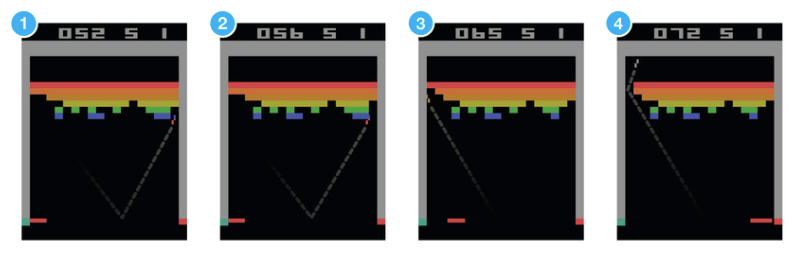如果想要让神经网络学着玩这个游戏。网络输入将会是屏幕图片，网络输出将会是三个动作：左移，右移，发射(球)。我们可以将其视为一个分类问题 - 每一帧画面，我们都必须做出决定：左移，右移还是发射。

尽管听起来比较简单，但我们需要大量的训练数据。当然，我们可以通过记录职业玩家的玩法来获得训练数据，但那不是机器真正该有的学习方式。

我们不需要谁成千上万次地来告诉机器每一帧的动作该怎么决定，我们所需要的仅仅是让机器在做了正确的决定后获得适当的反馈(reward), 然后让机器自己去领悟其余的东西。

这就是强化学习尽力在完成的任务。强化学习的地位处于监督学习和无监督学习之间。尽管监督学习给每条训练数据都标注了类别，而无监督学习什么类别也没标注，但是强化学习却给了稀疏的并且时间上有延迟的标注 - 奖励反馈(reward)。全靠这些奖励反馈，强化学习中的agent才能学习如何在环境中行动。

尽管这种思想比较符合咱们的直觉，但是实践中还是面临着许多挑战。还是拿打砖块游戏举例，当机器打掉了一块转，然后得分增加了，这常常与得分以前的最后几个动作没啥关系，而是与很多步以前的动作(比如你调整底部挡板的位置的动作)有更大的关系。

这个问题叫credit assignment problem(信用分配问题?) - 要确定某次得分与之前哪些动作有较为直接的关系，是一个比较难的问题。

一旦机器找到了一种获得奖励反馈的策略以后，是应该一直坚持它还是尝试一些别的路子来获得更大的奖励反馈呢？

这个问题叫explore-exploit dilemma(探索-开发困境) - 要一直采用已知的可行策略还是探索一些新的可能会更好的策略呢？

强化学习是动物学习过程中所采用的一种重要模型：爸妈的表扬，学校的成绩，工作中的薪水 - 这些都是奖励反馈的实例。

信用分配问题和探索-开发困境也都是我们每天都面临着的问题，这也是为什么要探索强化学习的原因；在这个探索过程中，游戏为我们提供了一个很棒的找到新方法的沙盒世界。

2 马尔科夫过程

现在我们的问题是该如何形式化一个强化学习的问题，最常用的方法将其表述为一个马尔科夫过程。

假如(你可以脑补打砖块游戏)环境中有一个agent能执行某些特定操作(i.e.左移木板，右移木板，发射球)，这些操作有时(当游戏处于某些特定状态)能给agent带来奖励反馈；每个操作都会改变这个环境，并将环境带入一个新的状态；在新的状态中，agent又将继续执行操作，以此类推下去。

在此过程中，agent在选择操作时所形成的规律/准则就叫策略(policy)；环境是随机的，这也意味着操作执行后到达的下一个状态也是随机的。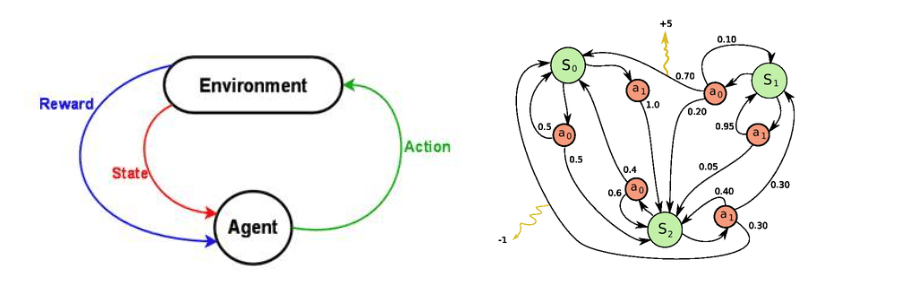马尔科夫决策过程就是这三者组成：1) 环境可能出现的状态(state)，2) agent所能执行的操作(action)以及3) 指导在所有状态下具体该如何执行操作的策略(policy)。

一段完整的马尔科夫过程会形成一段状态，动作和奖励反馈所构成的有限序列。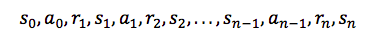上图中si$s_i$表示状态，ai$a_i$表示操作，ri+1$r_{i+1}$表示操作执行后得到的奖励反馈。sn$s_n$是这段马尔科夫过程的结束状态。值得一提的是，马尔科夫过程有一个假设前提：si+1$s_{i+1}$仅仅由si$s_i$和ai$a_i$联合决定，之前的状态和动作则完全不起作用。

3 折扣未来奖励 Discounted Future Reward

为了在长期过程中得到最优的结果，我们不仅需要考虑即时的奖励反馈，也需要把未来的奖励反馈纳入考虑。

对于一段马尔科夫决策过程，整个过程中的反馈奖励用以下等式表示：

R=r1+r2+...+rnR = r_1+r_2+...+r_n

基于整个等式，从t$t$时刻到最终状态所得到的全部奖励反馈可以用以下等式表示：
Rt=rt+rt+1+...+rnR_t=r_t+r_{t+1}+...+r_n

但环境是随机的，我们就没法确定当我们下次做同样动作时是否还能得到同样的奖励反馈。而我们越接近未来，就越能确定未来，所以取而代之，我们采用折扣未来奖励这种做法(discounted future reward)：

Rt=rt+γrt+1+γ2rt+2+...+γn−trnR_t=r_t+γr_{t+1}+γ^2r_{t+2}+...+γ^{n-t}r_n

上式中，γ$γ$是0和1之间的折扣因子 - 越接近未来的奖励反馈，对t$t$时刻的奖励反馈的影响越小。可以看出，t$t$时刻的折扣未来奖励可以由t+1$t+1$时刻改写出来：

Rt=rt+γ(rt+1+γ(rt+2+...))=rt+γRt+1R_t = r_t+γ(r_{t+1}+γ(r_{t+2}+...))=r_t+γR_{t+1}

如果我们将γ$γ$设为0，那么得到的策略将非常“短视”，因为我们只考虑即时的奖励反馈。如果我们想要在即时奖励反馈和未来奖励反馈这两者间取得平衡，那么我们就应该将γ因子设为0.9之类的。当然，如果环境是确定的，所有同样的动作总是能导致同样的奖励反馈，那就可以把γ设为1了。

总的来说，一个好的策略总是能帮助agent获得最多的未来奖励反馈。

4 价值函数 Value function

除非整个过程结束，否则我们无法得到每个状态对应的奖励反馈；因此，引入了价值函数Value Function, v(s)$v(s)$来表示某个状态未来的潜在价值。

从定义上看，价值函数就是回报的期望：v(s)=E[Rt|St=s]v(s) = \mathbf {E} [R_t|S_t = s]有了价值函数，就有三种方法获取最优的策略Policy：

直接优化策略π(a|s)$\pi(a|s)$或者a=π(s)$a = \pi(s)$使得回报更高
通过估计价值函数来间接得到优化的策略 - 既然知道每一种状态的优劣，那么就知道应该怎么选择了，而这种选择就是策略 - DQN就是通过估计价值函数来实现的
第三种做法是融合上面的两种方法的actor-critic算法

5 贝尔曼方程 Bellman Equation

在折扣未来奖励话题中，将t$t$时刻的奖励反馈R$R$定义为
Rt=rt+γ(rt+1+γ(rt+2+...))=rt+γRt+1R_t = r_t+γ(r_{t+1}+γ(r_{t+2}+...))=r_t+γR_{t+1}

在价值函数话题中，将价值函数v(s)$v(s)$定义为
v(s)=E[Rt|St=s]v(s) = \mathbf {E}[R_t|S_t = s]

两个式子结合后，可以得到
v(s)=E[rt+γRt+1|St=s]v(s)=\mathbf {E}[r_t+γR_{t+1}|S_t = s]
=E[rt+γv(St+1)|St=s]= \mathbf {E}[r_t+γv(S_{t+1})|S_t = s]

上面这个公式就是贝尔曼方程的基本形态 - 它表明当前状态的奖励反馈由当前的奖励反馈和下一步的奖励反馈决定。

同时，它也表明价值函数是可以通过迭代来计算得出的。

6 动作值函数 Action-Value function

由于每个状态之后有多种动作可以选择，每个动作之后的状态又不一样，实际情况中，我们更关心在某个状态下的不同动作的价值，这就是动作值函数Qπ(s,a)$Q^\pi(s,a)$。

因为知道了某个状态下每个动作的价值，那么就可以选择价值最大的一个动作去执行了。

跟价值函数一样，动作值函数也是用奖励反馈来表示，但动作值函数的奖励反馈和价值函数的奖励反馈不一样：动作值函数是执行完动作at$a_t$之后得到的奖励反馈，价值函数是多种动作对应的奖励反馈的期望值。

显然，动作值函数更直观，也更容易理解。

有了上面的定义，动作值函数可以表示为：
Qπ(s,a)=E[rt+1+λrt+2+λ2rt+3+...|s,a]=Es′[r+λQπ(s′,a′)|s,a]\begin{align} Q^\pi(s,a) & = \mathbf E[r_{t+1} + \lambda r_{t+2} + \lambda^2r_{t+3} + ... |s,a] \\\\ & = \mathbf E_{s^\prime}[r+\lambda Q^\pi(s^\prime,a^\prime)|s,a] \end{align}

注：动作值函数加了π$\pi$意味着在策略π$\pi$下的动作价值，如果定义vπ(s)$v^\pi(s)$则表示在策略π$\pi$下的价值函数。

能计算动作值函数还不够，因为我们需要的是最优策略 - 这可以由动作值函数来得到。

最优动作值函数和一般动作值函数的关系：
Q∗(s,a)=maxπQπ(s,a)Q^*(s,a) = \max_\pi Q^\pi(s,a)

也就是说，最优动作值函数就是所有策略下的动作值函数的最大值。

这样的定义能让最优的动作价值具有唯一性，从而可以求解整个MDP。

那么套用上一节得到的value function，可以得到
Q∗(s,a)=Es′[r+λmaxa′Q∗(s′,a′)|s,a]Q^*(s,a) = \mathbf E_{s^\prime}[r+\lambda \max _{a^\prime}Q^*(s^\prime,a^\prime)|s,a]

因为最优的Q值必然为最大值，所以，等式右侧的Q值必然为使a′取最大的Q值。

下面介绍基于Bellman方程的两个最基本的算法，策略迭代和值迭代。

策略迭代 Policy Iteration

值迭代 Value Iteration

7 Q-learning

Q-Learning的思想根据值迭代得到。

值迭代每次都对所有的Q值更新一遍，也就是所有的状态和动作。但在实际情况下我们没办法遍历所有的状态和动作，我们只能得到有限的系列样本。

因此，只能使用有限的样本进行操作。

在Q-learning中，我们定义了函数Q(s,a)$Q(s,a)$，用以表示在s$s$状态执行a$a$操作时所能得到的最大的折扣未来奖励反馈。

Q(st,at)=maxRt+1Q(s_t,a_t)=max R_{t+1}

关于Q(s,a)$Q(s,a)$，可以描述为在状态s$s$执行动作a$a$后，在游戏结束时得到的最好分数。之所以叫Q函数，因为它指代的是“某个状态下执行某个动作”的这个决定的质量如何。

如果我们仅仅知道当前状态和动作，不知道那之后的一系列状态和动作集合，我们没办法评估出当前动作的最终得分。我们能做的，仅仅是假设“Q函数是存在的”。(=，=???)

当我们在玩游戏时，面临该执行A还是B操作，如果我们有一个Q函数，那我们会直接执行最高Q值的操作。

π(s)=argmaxaQ(s,a)π(s)=arg max_aQ(s,a)

其中π$π$代表策略，指导agent在某种状态下如何选择action。

现在问题转化为了如何找出Q函数。

先来看这个转移动作<s,a,r,s′>$$，正如之前提到的折扣未来奖励反馈一样，我们能用状态s$s$和动作a$a$的Q值来表示下一个状态s′$s'$的Q值：

Q(s,a)=r+γmaxa′Q(s′,a′)Q(s,a) = r + γmax_{a'}Q(s',a')

上式是Bellman 方程：当前状态下当前动作的最大未来奖励反馈等于当前动作的即时奖励反馈+下一状态的最大未来奖励反馈。(有点像踢皮球？现在搞不定的，就留给以后来解决。其实是动态规划…)

Q-learning的核心思想是通过Bellman方程来迭代逼近Q函数：
Q(St,At)←Q(St,At)+α(Rt+1+λmaxaQ(St+1,a)−Q(St,At))Q(S_{t},A_{t}) \leftarrow Q(S_{t},A_{t})+\alpha({R_{t+1}+\lambda \max _aQ(S_{t+1},a)} - Q(S_t,A_t))

虽然根据值迭代来计算出target Q值，但是这里并没有直接将这个Q值(估计出来的)直接赋予新的Q，而是采用渐进的方式类似梯度下降，朝target迈近一小步，取决于α,这就能够减少估计误差造成的影响。类似随机梯度下降，最后可以收敛到最优的Q值。

具体的算法如下：

最简单地，Q函数可以用一张表格来实现，表格的行头为状态，列头为动作。Q-learning算法可以见以下：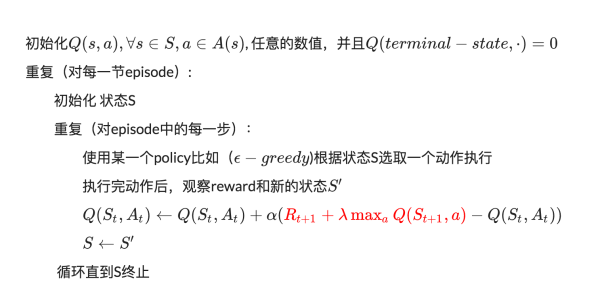算法中的α$α$是学习率，用以新得到的Q值能多大程度地修改以往的Q值，当α$α$=1,等式就和Bellman方程一样了。

等式中我们用来更新Q[St,At]$Q[S_t,A_t]$的maxaQ[st+1,a]$max_{a}Q[s_{t+1},a]$只是一种近似，甚至在学习的早期阶段，很可能完全就是错的。当随着迭代次数的上升，这种逼近会越来越准确，以至于当迭代到一定次数时，Q函数会收敛，并且反映出真正的Q值。

举个栗子：

为了得到最优策略Policy，我们会估算每一个状态下的每一种动作的价值Value。同时，每一个时间点的Q(s,a)和当前得到的Reward以及下一个时间点的Q(s,a)有关。

但是在实验中，只可能知道当前时刻的Q值，怎么知道下一个时刻的Q值呢？

Q-Learning建立在虚拟环境下无限次的实验。

这意味着可以把上一次实验计算得到的Q值拿来使用。这样就可以根据当前的Reward及上一次实验中记录的下一个时间片的Q值来更新当前的Q值了。

首先确定存储Q值的方式 - 最简单的想法是用矩阵，一个s一个a对应一个Q值，所以可以把Q值想象为一个很大的表格，横列代表s，纵列代表a，里面的数字代表Q值，如下表示：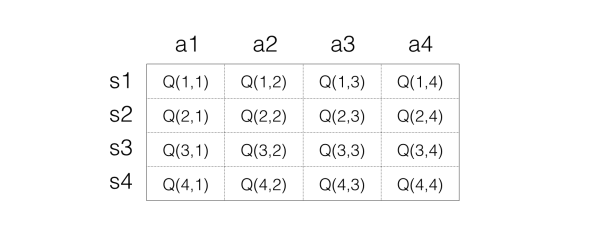接下来进行反复实验以更新表格。

Step 1：初始化Q矩阵，比如都设置为0.

Step 2：开始实验。根据当前Q矩阵及ϵ−greedy$\epsilon-greedy$方法获取动作。比如当前处在状态s1，那么在s1一列每一个Q值都是0，那么这个时候随便选择都可以。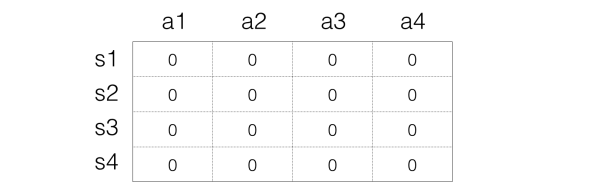假设选择a2动作，然后得到的reward是1，并且进入到s3状态，接下来要根据以下等式更新来Q值：
Q(St,At)←Q(St,At)+α(Rt+1+λmaxaQ(St+1,a)−Q(St,At))Q(S_{t},A_{t}) \leftarrow Q(S_{t},A_{t})+\alpha({R_{t+1}+\lambda \max _aQ(S_{t+1},a)} - Q(S_t,A_t))

假设α$\alpha$是1，λ$\lambda$也等于1，也就是每一次都把目标Q值赋给Q。那么这里公式变成：
Q(St,At)=Rt+1+maxaQ(St+1,a)Q(S_t,A_t) = R_{t+1} + \max_a Q(S_{t+1},a)

将此处的状态带入，得到
Q(s1,a2)=1+maxaQ(s3,a)Q(s_1,a_2) = 1 + \max_a Q(s_3,a)

注意：此时s3状态对应的各个动作，回报值最大为0，所以Q(s1,a2)=1+0=1$Q(s_1,a_2) = 1 + 0 = 1$,Q表格就变成：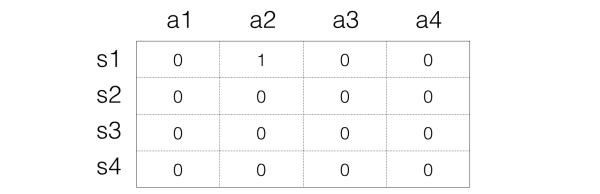Step 3：接下来进入下一次动作，现在处于状态s3，假设选择动作a3，然后得到1的reward，状态变成s1，此时s1状态对应的各个动作，回报值最大为0，那么我们同样进行更新：
Q(s3,a3)=2+maxaQ(s1,a)=2+1=3Q(s_3,a_3) = 2 + \max_a Q(s_1,a) = 2 + 1 = 3

所以Q的表格就变成：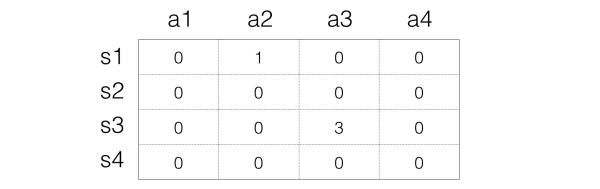Step 4： 反复进行上面的步骤，Q值也就在实验进行的同时反复更新。直到最后收敛。

8 Deep Q Network

打砖块游戏中，环境能够用木板的位置，球的位置和方向以及所有砖块的位置来定义。这种符合直觉的表征方式会随着游戏的改变而不再适用。如果想要找到更加通用的表征方式呢？

那就是屏幕像素。屏幕像素隐含着游戏中的所有相关信息，包括(打砖块中的)球的速度和方向等等(可以通过2张连续的帧计算出来)。

如果要应用DeepMind在论文《Human-level_control_through_deep_reinforcement_learning》中的预处理手段：取当前帧向前推4步得到的连续4帧，resize为84*84大小，转化为256阶的灰度图 - 这种方式下的打砖块游戏有25684×84×4≈1067970$256^{84×84×4}≈ 10^{67970}$种可能的游戏状态。

这时的Q表格中就有1067970$10^{67970}$行 - 比已知宇宙中的原子数目还多。尽管在这之中，有些状态并不会出现，这会是一张稀疏表格。很多状态几乎就不会被触发，即使如此，也要花费宇宙生命那么长的时间来使Q表格收敛，这被称为维度灾难(curse of dimensionality)。

那么，如果能够对之前没有出现过的一些状态的Q值进行近似猜测就很棒了。

简单来说，就是用一个函数来表示Q(s,a)：
Q(s,a)=f(s,a)Q(s,a) = f(s,a)

f$f$可以是任意类型的函数，如线性函数：

Q(s,a)=w1s+w2a+bQ(s,a) = w_1s + w_2a + b

其中w1,w2,b$w_1,w_2,b$是函数f$f$的参数，通过函数表示，无所谓s$s$到底是多大的维度，最后都能通过矩阵运算降维输出为单值的Q。

这是函数近似的基本思路。

如果我们就用w$w$来统一表示函数f的参数，那么就有
Q(s,a)=f(s,a,w)Q(s,a) = f(s,a,w)

之所以叫作近似，是因为我们并不知道Q值的实际分布情况，本质上是用一个函数来近似Q值的分布，所以也可以说是
Q(s,a)≈f(s,a,w)Q(s,a)\approx f(s,a,w)

而这就是深度学习的切入点。

神经网络能够将高度结构化数据的特征提取出来。那我们可以将Q函数用神经网络的形式表征出来 - 以状态和动作为输入，然后输出相应的Q值。或者我们可以仅仅将状态作为输入，然后输出可能发生的每个动作的Q值。

这种思路的优点在于，如果我们想执行Q值更新或者执行具有最高Q值的操作的话，只需要做一次网络前传，然后就能立即得到所有可能动作的Q值了。

对于Atari游戏而言，这是一个高维状态输入(原始图像)，低维动作输出(几个离散的动作，比如上下左右)的过程。在表示函数时，我们只需要对高维状态进行降维，而不需要对动作也进行降维处理。

Q(s)≈f(s,w)$Q(s) \approx f(s,w)$，只把状态s作为输入，但是输出的时候输出每一个动作的Q值，也就是输出一个向量[Q(s,a1),Q(s,a2),Q(s,a3),...,Q(s,an)]$[Q(s,a_1),Q(s,a_2),Q(s,a_3),...,Q(s,a_n)]$，这里的输出是一个包含了所有动作的Q值的向量。只要输入状态s，同时可以得到所有的动作Q值，可以更方便的进行Q-Learning中动作的选择与Q值更新。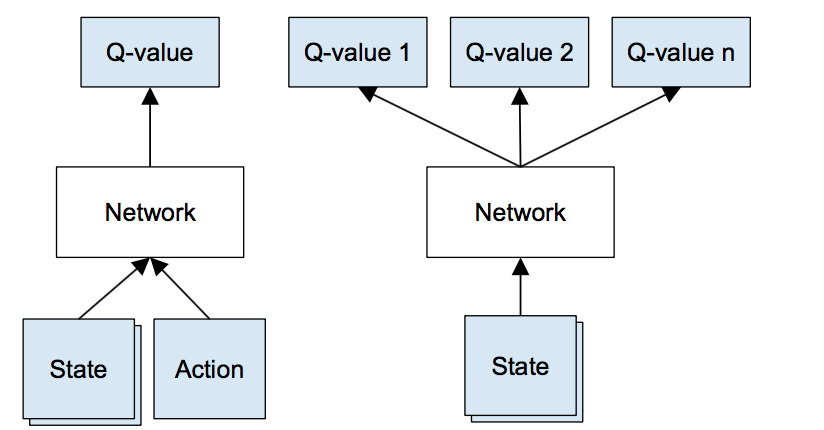Left: Naive formulation of deep Q-network. Right: More optimized architecture of deep Q-network, used in DeepMind paper.

DeepMind在上面那篇论文中用的网络结构如下：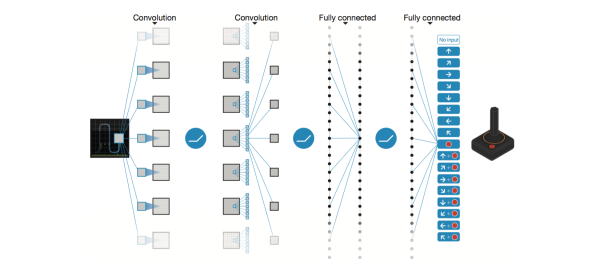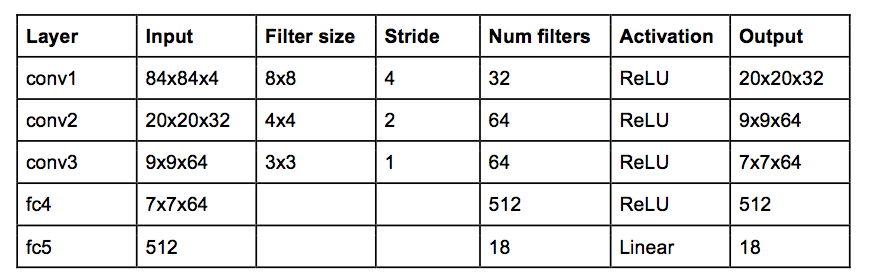这就是传统的CNN结构，但是没pooling层 - pooling层的平移不变特性将会让网络对图片中物体的位置改变不再敏感 - 而(打砖块)游戏中，球的位置是对于奖励反馈的确定是十分重要的，所以不能有pooling层。

网络输入为4张连续的84*84的游戏画面灰度图，网络输出为每个可能动作的Q值，Q值可以为任何实数值，这就转化成了回归任务，可以用平方差loss进行优化。

神经网络的训练是一个最优化问题，最优化某个损失函数loss function，也就是标签和网络输出的偏差，目标是让损失函数最小化。为此，我们需要有样本，巨量的有标签数据，然后通过反向传播使用梯度下降的方法来更新神经网络的参数。

所以要训练Q网络，我们要能够为Q网络提供有标签的样本。

如何为Q网络提供有标签的样本？

答案就是Q-Learning算法。

回忆Q-Learning算法，Q值的更新依靠的是利用Reward和Q计算出来的目标Q值：
Rt+1+λmaxaQ(St+1,a)R_{t+1}+\lambda \max _aQ(S_{t+1},a)

因此，我们可以把target Q值作为标签，我们的目标就是让Q值趋近于target Q值。

因此，Q网络训练的损失函数就是上面公式是s‘,a‘$s^,a^$即下一个状态和动作。

考虑一个转移动作<s,a,r,s′>$< s, a, r, s'>$，以前的算法中的Q值更新规则需要做以下调整：

当前状态s$s$在网络中进行前传后，得到所有可能动作的Q值预测；
下一状态s′$s'$在网络中进行前传后，计算所有输出中最大值maxa′Q(s′,a′)$max_{a'}Q(s',a')$;
将动作的目标Q值表示为r+γmaxa′Q(s′,a′)$r + γmax_{a'} Q(s', a')$(即上一步中计算出来的最大值)，同时将其他动作的目标Q值设为和第一步中返回的值相等，让所有的输出的误差为0；
用反向传播进行网络权重更新

既然确定了损失函数，即cost，也确定了获取样本的方法。那么DQN的整个算法也就成型了！

9 经验重放 Experience Replay

目前为止，我们大概知道了如何用Q-learning和CNN的近似Q函数来评估每个状态的未来奖励反馈，然而实验表明，非线性函数的Q值逼近非常不稳定，必须得用很多trick来帮助其收敛，同时耗时巨大。

Experience Replay就是这样的一种技术，在游戏数据的采集过程中，所有的经验数据<s,a,r,s′>$< s, a, r, s'>$都被存储到一个回放池(replay memory)中。当训练网络时，从回放池中随机地取一小撮数据，而不是最新连续的几条转换数据，进行训练。

这种方法打破了连续数据之间的关联性。由于神经网络是有监督模型，所以要求训练数据必须是独立同分布的，否则将会使得网络陷于局部最小值。

同时，这种经验回放简化了算法的调试和测试，让训练更像有监督学习。毕竟，我们可以从人类玩家那收集到这些经验数据然后用于网络训练。

10 探索-开发 Exploration-Exploitation

在Q-Learning算法中，需要使用某一个policy来生成动作，这个policy不是优化的那个policy，所以Q-Learning算法叫做Off-policy的算法；另一方面，因为Q-Learning完全不考虑model(就是环境的具体情况)，只考虑看到的环境及奖励反馈，因此是model-free的方法。

Q-learning尝试解决信用分配问题 - 将奖励反馈一直回传到做关键决定的时间节点，但是我们还得面临Exploration-Exploitation困境。

在Q-Learning中，我们需要根据哪种policy来生成action呢？有两种做法：

随机的生成一个动作
根据当前的Q值计算出一个最优的动作，这个策略 π$\pi$称之为greedy policy贪婪策略。也就是
π(St+1)=argmaxaQ(St+1,a)\pi(S_{t+1}) = arg\max _aQ(S_{t+1},a)

使用随机的动作就是exploration，也就是探索未知的动作会产生的效果，有利于更新Q值，获得更好的policy。而使用greedy policy也就是target policy则是exploitation，利用policy，相对来说就不好更新出更好的Q值，但可以得到更好的测试效果用于判断算法是否有效。

将两者结合起来就是所谓的ε−greedy$ε-greedy$探索 - 设置因子ε$ε$，每次执行动作时，有ε的几率执行随机动作(剩下1-ε的几率执行具有最高Q值的动作)。DeepMind实际上让ε随着时间从1逐渐减少到0.1 - 最开始时，agent执行完全随机的动作，以此探索最大化的状态空间，然后逐渐将“探索”降至一个固定的频率。可以更改\epsilon的值从而得到不同的exploration和exploitation的比例。

这里需要说明的一点是使用\epsilon-greedy策略是一种极其简单粗暴的方法，对于一些复杂的任务采用这种方法来探索未知空间是不可取的。因此，最近有越来越多的方法来改进这种探索机制。

11 DQN训练

以下就是带有经验回放的最终的深度强化学习算法：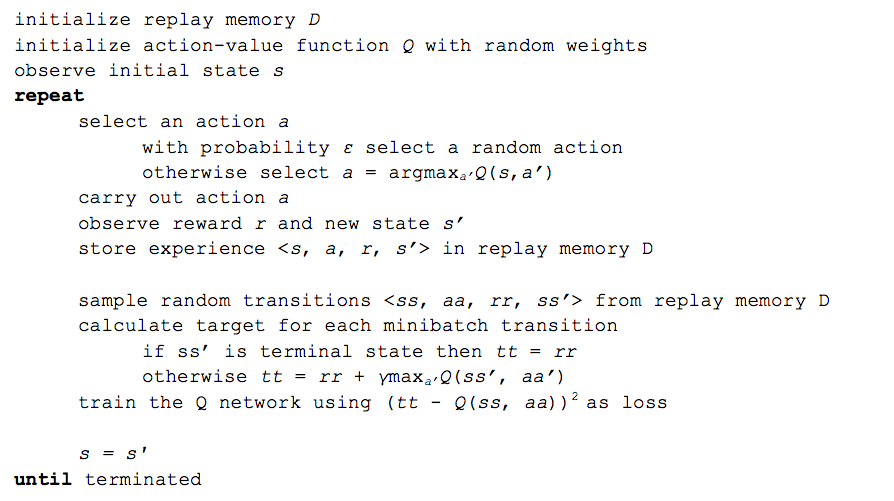两种表述方式，自取。算法看起来很长，其实就是在反复试验，然后存储数据。当数据存到一定程度，就每次随机采用数据，进行梯度下降。

在DQN中增强学习Q-Learning算法和深度学习的SGD训练是同步进行的，通过Q-Learning获取无限量的训练样本，然后对神经网络进行训练。

而样本的获取关键是计算y，也就是标签。

当然，DeepMind实际过程中还运用了很多其他的trick来让算法更有效(target network, error clipping, reward clipping 等等)

深度强化学习算法的惊艳点在于，它能学习任何东西。Q函数最初是随机的，最初的决策也基本就是“垃圾输出”；然后我们不断地利用它的“垃圾输出”进行网络的更新，再适时辅以奖励反馈，最后它就学会了这一切？？

更进一步

12 on-policy vs. off-policy

这是强化学习中的一种方法划分方式。

估计policy(value-function)的时候，需要用到一些样本，这些样本也需要采用某种策略(可能固定、可能完全随机、也可能隔一段时间调整一次)生成的。
而判断on-policy和off-policy的关键在于，你所估计的policy(value-function)  和 生成样本所采用的policy  是不是一样的。如果一样，那就是on-policy的，否则是off-policy的。

on-policy: 生成样本的policy跟网络更新参数时使用的policy相同。

典型为SARAS算法，执行两次动作得到(s,a,r,s’,a’)才可以更新一次；而且a’是在特定π的指导下执行的动作，因此估计出来的Q(s,a)是在该π之下的Q-value，即Q_π(s,a)，因此生成样本的policy和学习时的policy相同，算法为on-policy算法。该方法会遭遇探索-开发的矛盾，只利用目前已知的最优选择，可能学不到最优解，收敛到局部最优，而加入探索又降低了学习效率。epsilon-greedy 算法是这种矛盾的折衷。优点是直接了当，速度快，劣势是不一定找到最优策略。
off-policy：生成样本的policy跟网络更新参数时使用的policy不同。

典型为Q-learning算法，每次只需要执行一次动作得到(s,a,r,s’)就可以更新一次；因为a’永远是最优的那个action，因此你估计的策略应该是最优的，即Q_π*(s,a)，而你生成样本时用的策略则不一定是最优的，因此这里生成样本的policy和学习时的policy不同，为off-policy算法。先产生某概率分布下的大量行为数据(behavior policy)，意在探索。从这些偏离最优策略的数据中寻求target policy。当然这么做是需要满足数学条件的：假設π是目标策略, µ是行为策略，那么从µ学到π的条件是：π(a|s) > 0 必然有 µ(a|s) > 0成立。
两种学习策略的关系是：on-policy是off-policy 的特殊情形，其target policy 和behavior policy是一个。劣势是曲折，收敛慢，但优势是更为强大和通用。其强大是因为它确保了数据全面性，所有行为都能覆盖。

13 model-based vs. model-free

另一种强化学习中的方法划分方式。

Model-based: 尝试给环境建模，最终基于这个环境选择最优的策略。建模时一些微小的错误将给agent在选择动作时产生比较大的负面影响。
Model-free: 不对环境进行建模，而是一直在每一步中去尝试学习最优的策略，在多次迭代后就得到了整个环境最优的策略(e.g. Q-learning)。

14 RL两大分支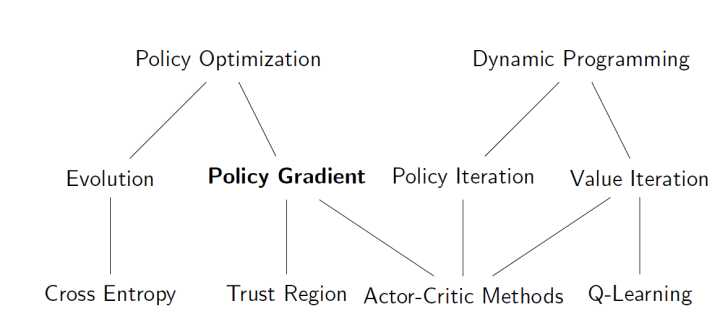可以看到，Q Learning 和 Policy Gradient分属RL的两大分支：Dynamic Programming 和 Policy Optimization。

P.S. Cross Entropy Method 是较为简单且经典的Policy Optimization 算法，在一些简单场景效果很好。

本篇博客主要整理自以下文章
《Guest Post (Part I): Demystifying Deep Reinforcement Learning》
《知乎专栏 - 智能单元》
强化学习中on-policy 与off-policy有什么区别？
增强学习中的on-policy和off-policy的区别
强化学习on-policy跟off-policy的区别
解释model-based和model-free，on-policy和off-policy区别？
reinforcement learning，增强学习：Integrating Learning and Planning
展开全文DQN 马尔科夫过程 贝尔曼方程
• 深度增强学习（Deep reinforcement learning, DRL）是DeepMind（后被谷歌收购）近几近来重点研究且发扬光大的机器学习算法框架。两篇Nature上的奠基性论文（DQN和AlphaGo）使得DRL这一深度学习（Deep learning, DL）...DQN AlphaGo
• 而其中的深度增强学习算法是AlphaGo的核心，也是通用人工智能的实现关键。本文将带领大家了解深度增强学习的前沿算法思想，领略人工智能的核心奥秘。 前言 深度增强学习（Deep Reinforcement Learning
• 之前写过该项目的环境setup介绍《常用增强学习实验环境 I (MuJoCo, OpenAI Gym, rllab, DeepMind Lab, TORCS, PySC2)》以及其中的另一重要算法-PPO算法走读《深度增强学习PPO（Proximal Policy Optimization）算...DDPG baselines
• 深度增强学习入门) PDF VIDEODeep Reinforcement Learning 深度增强学习是一套很复杂的方法，在这里只了解皮毛。 Scenario of Reinforcement Learning 有个傻傻的机器人小白(Agent)去闯荡世界(Environment)，
• 深度增强学习：走向通用人工智能之路 本文是系列文章中的第一篇，是对深度增强学习/深度强化学习的基本介绍以及对实现通用人工智能的探讨。 现在但凡写人工智能的文章，必提Alpha Go。也正是因为Alpha Go在围棋...人工智能
• 之前在文章《深度增强学习（DRL）漫谈 - 从DQN到AlphaGo》扯了一些关于DRL的内容，但因为是以DQN为主线，其中大部分谈的是value-based方法。我们知道传统增强学习（Reinforcement learning, RL）中除了value-baActor Critic
• 深度增强学习Deep Reinforcement Learning是将深度学习与增强学习结合起来从而实现从Perception感知到Action动作的端对端学习的一种全新的算法。简单的说，就是和人类一样，输入感知信息比如视觉，然后通过深度神
• 深度增强学习（Deep reinforcement learning, DRL）是DeepMind（后被谷歌收购）近几年来重点研究且发扬光大的机器学习算法框架。两篇Nature上的奠基性论文（DQN和AlphaGo）使得DRL这一深度学习（Deep learning,
• blog.floodsung.com主要内容将全面聚焦深度增强学习Deep Reinforcement Learning！使用Github Pages和Hexo。更简洁的界面是我转移博客的原因之一。新的blog将带给大家更好的阅读体验！新的文章也仍然会同步发布到...
• 深度增强学习Deep Reinforcement Learning是将深度学习与增强学习结合起来从而实现从Perception感知到Action动作的端对端学习的一种全新的算法。简单的说，就是和人类一样，输入感知信息比如视觉，然后通过深度神经...
• 从OpenAI看深度学习研究前沿OpenAI的三个主要研究方向 1. 深度生成模型 Deep Generative Model 通过学习现有的数据生成新的数据。 相关研究： 1）otoro.net 学习文字自动生成新的文字 2）Deep Dream Neural...
• Deep Reinforcement Learning深度增强学习可以说发源于2013年DeepMind的Playing Atari with Deep Reinforcement Learning 一文，之后2015年DeepMind 在Nature上发表了Human Level Control through ...
• ﻿﻿http://rll.berkeley.edu/deeprlcoursesp17/#lecture-videos人家的课程都共享出来了。。。真是不错。。。Global overview of Imitation Learning，再加一篇模仿学习的文章
• 视频链接：李宏毅机器学习(2016)_演讲•公开课_科技_bilibili_哔哩哔哩 课程资源：Hung-yi Lee 课程相关PPT已经打包命名好了：链接：https://pan.baidu.com/s/1c3Jyh6S 密码：77u5 我的第二十三讲...
• 强化学习学习一个最优策略(policy)，可以让 智能体(Agent) 在 特定环境(Environment) 中，根据当前状态(state)，做出行动(action)，从而获得最大回报(reward)。 DQN要做的就是将卷积神经网络（CN......